Courses
Courses for Kids
Free study material
Offline Centres
More

# NCERT Solutions for Class 8 Maths Chapter 15 - ExerciseLast updated date: 06th Dec 2023
Total views: 531k
Views today: 14.31k

## NCERT Solutions for Class 8 Maths Chapter 15 Introduction to Graphs (EX 15.3) Exercise 15.3

Free PDF download of NCERT Solutions for Class 8 Maths Chapter 15 Exercise 15.3 (EX 15.3) and all chapter exercises at one place prepared by expert teacher as per NCERT (CBSE) books guidelines. Class 8 Maths Chapter 15 Introduction to Graphs Exercise 15.3 Questions with Solutions to help you to revise complete Syllabus and Score More marks. Register and get all exercise solutions in your emails.

Vedantu also provides NCERT Solutions for all subjects and classes for free. Science Students who are looking for NCERT Solutions for Class 8 Science will also find the Solutions curated by our Master Teachers really Helpful.

## NCERT Solutions for Class 8 Maths Chapter 15 Introduction to Graphs

### Exercise 15.3

1. Draw the graphs for the following tables of values, with suitable scales on the axes:

(a) Cost of apples

 Number of apples 1 2 3 4 5 Cost(in Rs) 5 10 15 20 25

Ans: Take the number of apples on the x-axis and cost on the y-axis. Take scale for x-axis as ${\text{1 unit}} = 1{\text{ apple}}$ and for y-axis as ${\text{1 unit}} = 5{\text{ rupees}}$.

Now plot the points ${\text{(1,5),(2,10),(3,15),(4,20)}}$and ${\text{(5,25)}}$to get the number of apples-cost linear graph: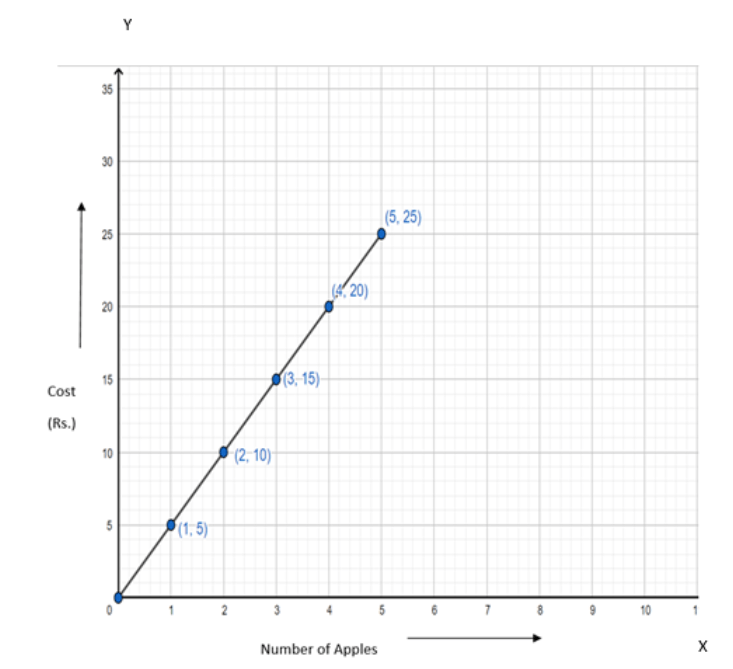(b) Distance travelled by a car

 Time(in hours) 6 a.m. 7 a.m. 8 a.m. 9 a.m. Distance(in km) 40 80 120 160

Ans: Take time on x-axis and distance travelled on  y-axis. Take scale for x-axis as ${\text{1 unit}} = 1{\text{ hour}}$and for y-axis as${\text{1 unit}} = 40{\text{ km}}$.

Now plot the points ${\text{(6,40),(7,80),(8,120)}}$and ${\text{(9,160)}}$to get the time-distance linear graph: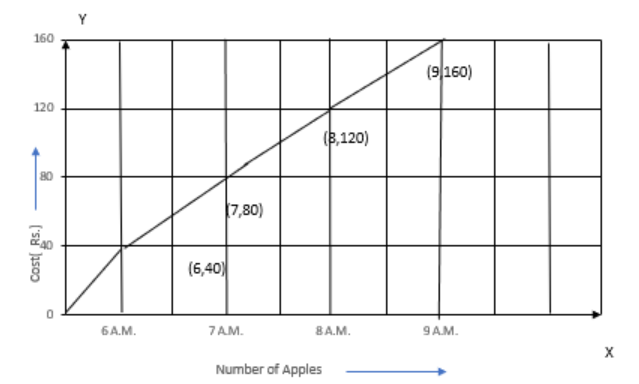(i) How much distance did the car cover during the period 7.30 a.m. to 8 a.m.?

Ans: ${\text{Distance travelled by 7:30 a}}{\text{.m}}{\text{. }} = 100{\text{ km}}$

${\text{Distance travelled by 8 a}}{\text{.m}}{\text{. }} = 120{\text{ km}}$

${\text{Distance travelled by the car during the period 7:30 a}}{\text{.m}}{\text{. to 8 a}}{\text{.m}}{\text{.}} = {\text{Distance travelled till 8 a}}{\text{.m}}{\text{.}} - {\text{Distance travelled till 7:30 a}}{\text{.m}}{\text{.}}$

${\text{Distance travelled by the car during the period 7:30 a}}{\text{.m}}{\text{. to 8 a}}{\text{.m}}{\text{.}} = 120{\text{ km}} - 100{\text{ km}}$

${\text{Distance travelled by the car during the period 7:30 a}}{\text{.m}}{\text{. to 8 a}}{\text{.m}}{\text{.}} = 20{\text{ km}}$

Thus, the car covers 20 km during the period 7:30 a.m. to 8 a.m.

(ii) What was the time when the car had covered a distance of 100 km since its start?

Ans: At 7:30 a.m. , the car had covered a distance of 100 km since it’s start.

(c) Interest on deposits for a year:

 Deposit(in Rs) 1000 2000 3000 4000 5000 Simple Interest(in Rs) 80 160 240 320 400

Ans: Take deposit on x-axis and simple interest on  y-axis. Take scale for x-axis as ${\text{1 unit}} = 1000{\text{ Rs}}$and for y-axis as${\text{1 unit}} = 80{\text{ Rs}}$.

Now plot the points ${\text{(1000,80),(2000,160),(3000,240),(4000,320)}}$and ${\text{(5000,400)}}$to get the time-distance linear graph: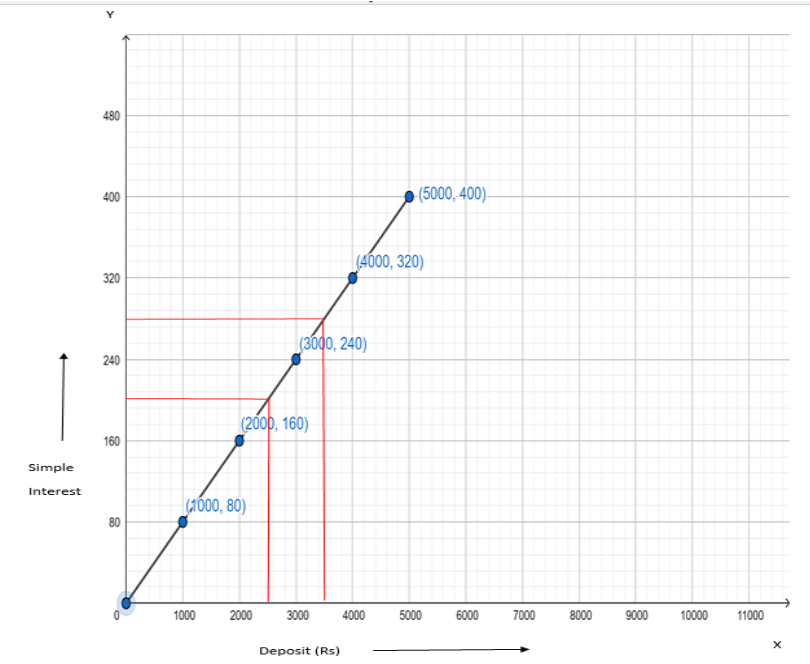(i) Does the graph pass through the origin?

Ans: From the graph, one can observe that the graph passes through the origin.

(ii) Use the graph to find the interest on Rs 2500 for a year.

Ans: Rs2500 lies in between Rs 2000 and Rs 3000, therefore interest on Rs 2500 lies right in the middle of Rs 160 and Rs 240, i.e., Rs 200.

Thus, interest on Rs 2500 for a year is 200.

(iii) To get an interest of Rs 280 per year, how much money should be deposited?

Ans: Rs 280 lies right in the middle of Rs 240 and Rs 320, therefore deposit with respect to it lies right in the middle of Rs 3000 and Rs 4000, i.e., Rs 3500.

Thus, to get an interest of Rs 280 per year, Rs 3500 must be deposited.

2. Draw a graph for the following:

(i)

 Sides of a square(in cm) 2 3 3.5 5 6 Perimeter(in cm) 8 12 14 20 24

Ans: Take sides of a square(in cm) on x-axis and perimeter(in cm) on  y-axis. Take scale for x-axis as ${\text{1 unit}} = 1{\text{ cm}}$and for y-axis as${\text{1 unit}} = 4{\text{ cm}}$.

Now plot the points ${\text{(2,8),(3,12),(3}}{\text{.5,14),(5,20)}}$and ${\text{(6,24)}}$to get the side-perimeter linear graph of a square: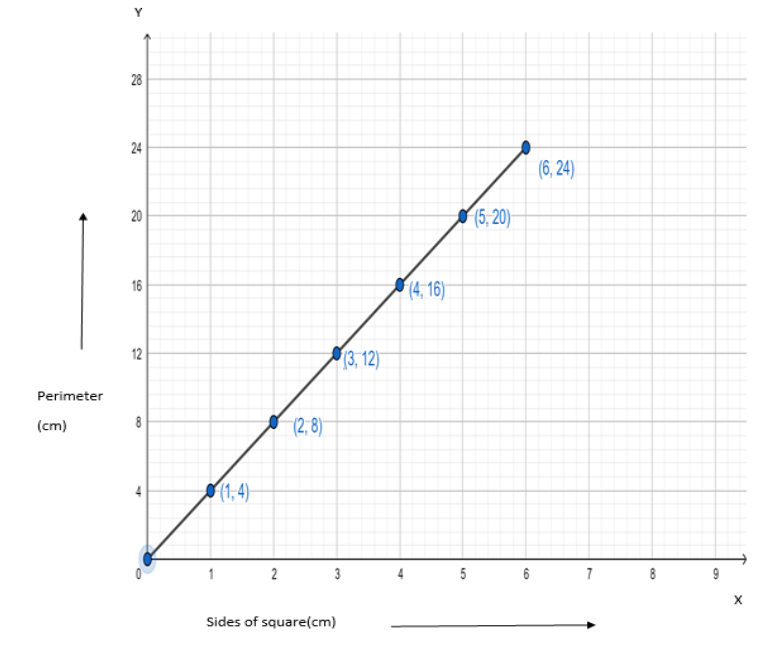Is it a linear graph?

Ans: The line  graph is a straight line, therefore it is a linear graph.

(ii)

 Sides of a square(in cm) 2 3 4 5 6 Area(in $\mathop {cm}\nolimits^2$) 4 9 16 25 36

Ans: Take sides of a square(in cm) on x-axis and area(in $c{m^2}$) on  y-axis. Take scale for x-axis as $1{\text{ unit}} = 1{\text{ cm}}$and for y-axis as$1{\text{ unit}} = 4{\text{ c}}{{\text{m}}^2}$.

Now plot the points ${\text{(2,4),(3,9),(4,16),(5,25)}}$and ${\text{(6,36)}}$to get the side-area linear graph of a square: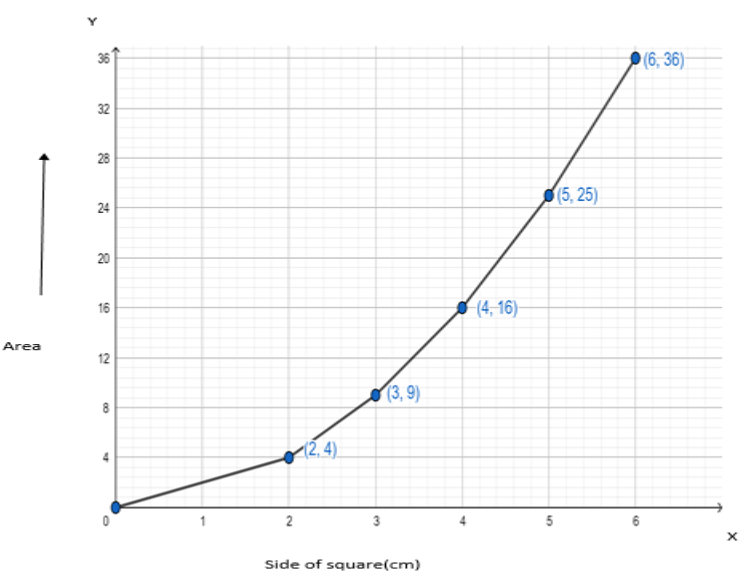Is it a linear graph?

Ans: The line graph is not a straight line, therefore it is not a linear graph.

### NCERT Solutions for Class 8 Maths Chapter 15 Introduction to Graphs Exercise 15.3

Opting for the NCERT solutions for Ex 15.3 Class 8 Maths is considered as the best option for the CBSE students when it comes to exam preparation. This chapter consists of many exercises. Out of which we have provided the Exercise 15.3 Class 8 Maths NCERT solutions on this page in PDF format. You can download this solution as per your convenience or you can study it directly from our website/ app online.

Vedantu in-house subject matter experts have solved the problems/ questions from the exercise with the utmost care and by following all the guidelines by CBSE. Class 8 students who are thorough with all the concepts from the Subject Introduction to Graphs textbook and quite well-versed with all the problems from the exercises given in it, then any student can easily score the highest possible marks in the final exam. With the help of this Class 8 Maths Chapter 15 Exercise 15.3 solutions, students can easily understand the pattern of questions that can be asked in the exam from this chapter and also learn the marks weightage of the chapter. So that they can prepare themselves accordingly for the final exam.

Besides these NCERT solutions for Class 8 Maths Chapter 15 Exercise 15.3, there are plenty of exercises in this chapter which contain innumerable questions as well. All these questions are solved/answered by our in-house subject experts as mentioned earlier. Hence all of these are bound to be of superior quality and anyone can refer to these during the time of exam preparation. In order to score the best possible marks in the class, it is really important to understand all the concepts of the textbooks and solve the problems from the exercises given next to it.

Do not delay any more. Download the NCERT solutions for Class 8 Maths Chapter 15 Exercise 15.3 from the Vedantu website now for better exam preparation. If you have the Vedantu app in your phone, you can download the same through the app as well. The best part of these solutions is these can be accessed both online and offline as well.

## FAQs on NCERT Solutions for Class 8 Maths Chapter 15 - Exercise

1. What information should a student have before starting Exercise 15.3, Chapter 15?

A student needs to be able to recall all of the significant properties that they learned earlier in this chapter. Due to their incomplete understanding of the material, students frequently become stuck on theorems and questions.

2. What are some of the most important questions from Exercise 15.3 chapter 15?

Some of the important questions from this Chapter 15 Exercise 15.3 are Q2 One of the most important questions in this exercise.

3. Why is doing Vedantu NCERT solutions for Class 8 Maths, Chapter 15, Exercise 15.3, Introduction to Graphs, important?

Vedantu experts have solved the questions from the exercise with the utmost care and by following all the guidelines provided by CBSE. Class 8 students who understand all of the concepts from the Areas of You textbook can easily score the highest possible marks in the final exam.

4. What is the summary of this chapter 15 exercise (15.3)?

This exercise, which is optional, is crucial for understanding graphs. There are only 2 questions, and the first one is extremely significant.

5. Why should I refer to Vedantu’s NCERT Solutions for class 8 Maths Chapter 15 Introduction to Graphs (EX 15.3) Exercise 15.3?

If you have the appropriate study materials, getting a perfect score in maths shouldn't be too difficult. For a subject like mathematics, studying just the chapters is never enough; therefore, students should practise the questions on their respective exercises. As per CBSE requirements, Vedantu's knowledgeable staff has prepared NCERT solutions. Detail and accuracy are used to explain the solutions. Your grades will go up and you'll be better prepared for competitive exams if you use quick problem-solving techniques. On the website and mobile app Vedantu, all of these solutions can be downloaded without charge.